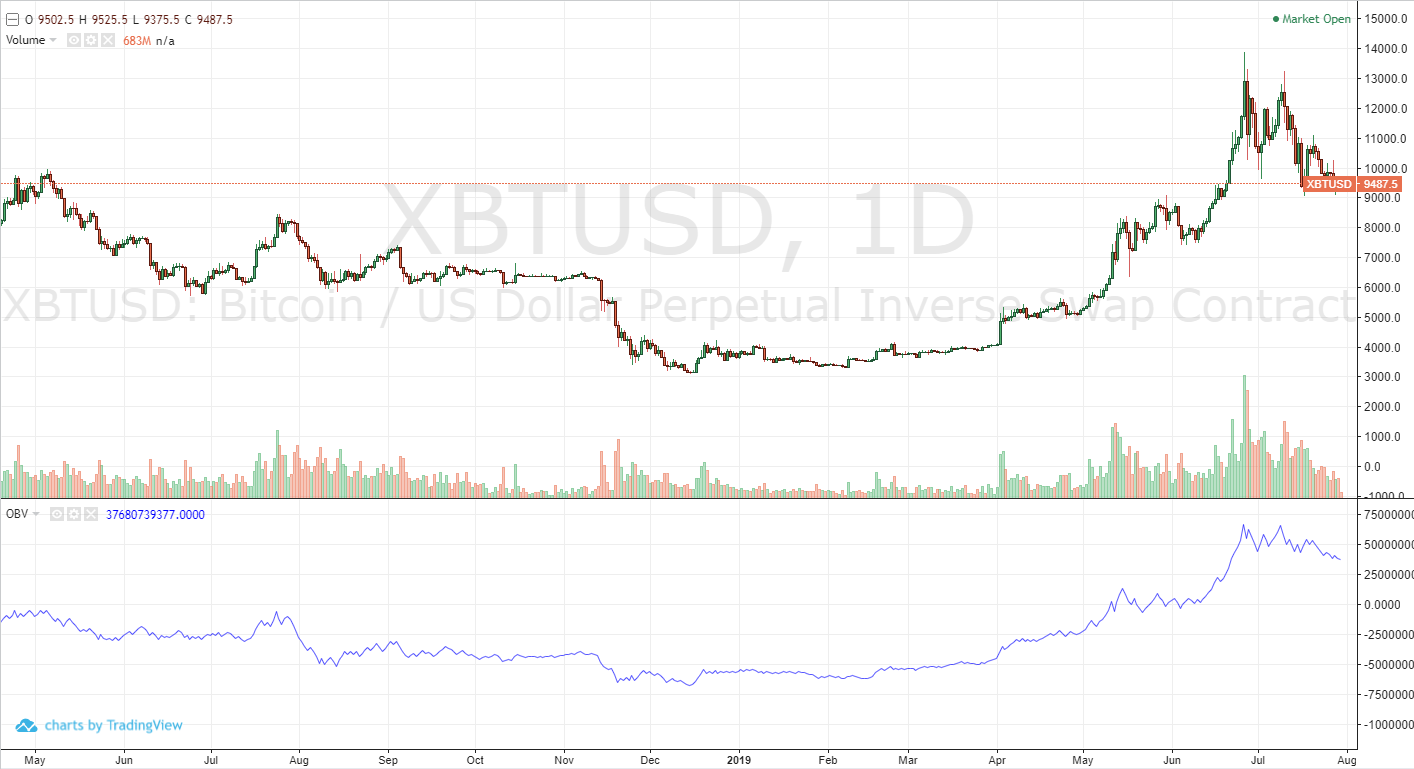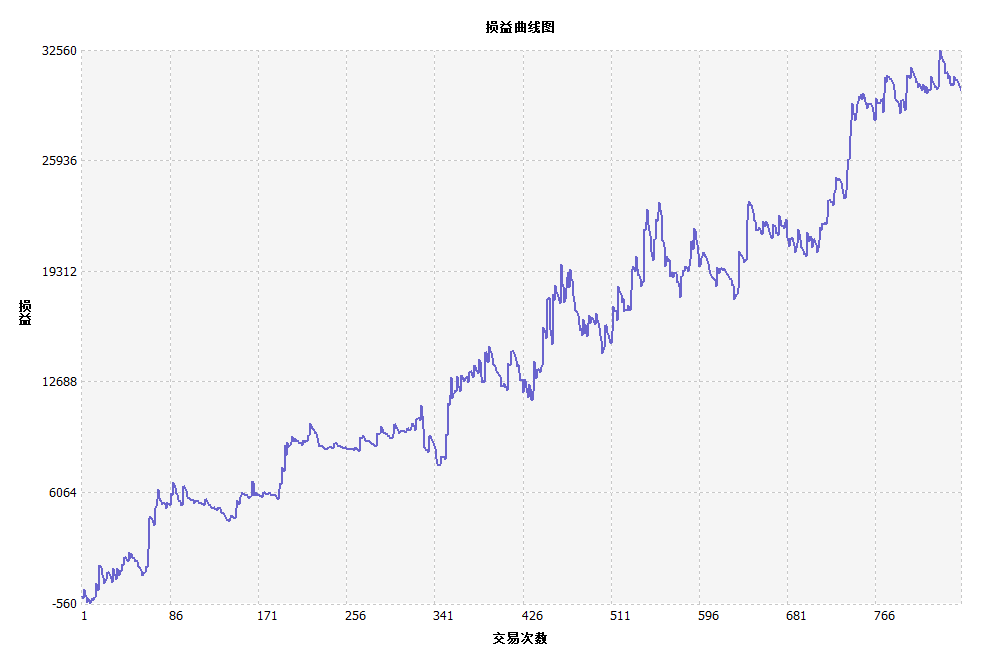2、图表中的能量潮4、能量潮的计算方法

• 如果当前K线收盘价大于上根K线收盘价，那么：
• OBV(i) = OBV(i - 1) + VOLUME(i)
• 如果当前K线收盘价小于上根K线收盘价，那么：
• OBV(i) = OBV(i - 1) - VOLUME(i)
• 如果当前K线收盘价等于上根K线收盘价，那么：
• OBV(i) = OBV(i - 1)

• OBV(i)：当前K线的能量潮
• OBV(i - 1)：上根K线的能量潮
• VOLUME(i)：当前K线的成交量

6、能量潮交易逻辑

• 首先计算出能量潮（OBV）
• 接着计算出真实波动幅度（ATR）
• 然后计算出两根能量潮的均线
• 多头开仓：两条均线向上，并且金叉
• 空头开仓，两条均线向下，并且死叉
• 多头平仓，两条均线向下，或者死叉
• 空头平仓，两条均线向上，或者金叉

7、基于能量潮的交易策略代码

N:=10;
OBV:=SUM(IFELSE(CLOSE>REF(CLOSE,1),VOL,IFELSE(CLOSE<REF(CLOSE,1),-VOL,0)),0);
TR:=MAX(MAX((HIGH-LOW),ABS(REF(CLOSE,1)-HIGH)),ABS(REF(CLOSE,1)-LOW));
ATR:=MA(TR,N);
B:EMA2(OBV*ATR,N);
D:EMA2(OBV*ATR,N*2);
B>REF(B,1) && D>REF(D,1) && B>D,BK;
B<REF(B,1) || D<REF(D,1) || B<D,SP;
B<REF(B,1) && D<REF(D,1) && B<D,SK;
B>REF(B,1) || D>REF(D,1) || B>D,BP;
AUTOFILTER;

8、策略回测

• 交易品种：螺纹钢指数
• 时间：2009年03月27日~2019年07月31日
• 周期：一小时
• 滑点：开平仓各2跳
• 手续费交易所2倍